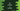# C# program to reverse a number## C# program to reverse a number:

In this post, we will learn how to reverse a number in C#. To reverse a number, we can use a loop, pick the rightmost digit on each iteration and add it to a different number.

### Algorithm to use:

To reverse a number, we can use a loop that picks the rightmost digit of the number and keeps it adding to a different reverse number.

For example, if we are reversing 123,

• Initialize reverse as 0.
• Pick the rightmost value of 123, i.e. 3, and add it to reverse. It will be 3. Change 123 to 12.
• Pick the rightmost value of 12, i.e. 2, add it to reverse. It will be 32. Change 12 to 1.
• Add 1 to the end of 32 i.e. it will be 321.

### C# program:

Below is the complete program that uses the above algorithm:

``````using System;

public class Program
{
public static void Main()
{
int num;
int reverse = 0;

Console.WriteLine("Enter the number : ");

while(num > 0){
reverse = reverse * 10 + num%10;
num = num/10;
}

Console.WriteLine("Reversed number : {0}",reverse);

}
}``````

Here,

• num is the number entered by the user.
• reverse is the reversed number i.e. reverse of num.
• It is reading the number and storing that in num
• The while loop is used to reverse the number. It keeps adding the rightmost digit of num to reverse. Once the while loop ends, reverse holds the reversed value of num.
• Finally, it is printing the value of the reversed number i.e. reverse.

### Sample output:

This program will print output as like below:

``````Enter the number :
1234
Reversed number : 4321

Enter the number :
6657678
Reversed number : 8767566``````

As you can see here, it reverses the number and prints that.